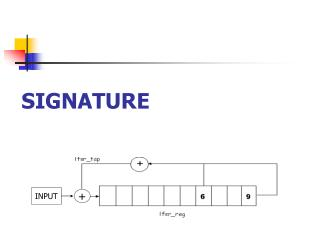DownloadDownload PresentationSIGNATURE

# SIGNATURE

Télécharger la présentation## SIGNATURE

- - - - - - - - - - - - - - - - - - - - - - - - - - - E N D - - - - - - - - - - - - - - - - - - - - - - - - - - -
##### Presentation Transcript

1. SIGNATURE INPUT +

2. PROPERTIES • Circuits signature gives us the ability to check Circuits - if they are undamaged. • Checking the output of the CUT vs. a known good response is inefficient and not practical. • Using Signature Analysis enables us to check CUT efficiently.

3. The math behind it… + P (x) … G (x) SIG. Reg. Q (x) + Initial State - I (x) = 0 Final State - R (x) G (x) R (x) ____ = Q (x) + ____ P (x) P (x) It Satisfies this polynomial equation :

4. The math behind it(2)… • M – Number of bits in stream (input) • N – Number of bits in Sig. Reg. • The Num. of streams that produces a specific sig. is 2M-N (= 2M / 2N ) • The Num. of bad streams that will yield good sig. is 2M-N - 1

5. The math behind it(3)… • When M>>N the probability for having an unnoticeable mistake is 2M-N - 1 2-N 2M - 1 ≈ • So, for as the Sig. Reg. is bigger we get a better approximation on the CUT

6. S-Edit (1_xor_6_SIG)

7. L-Edit (1_xor_6_SIG)

8. L-Edit(2) (1_xor_6_SIG)

9. S – Edit Simulation

10. S – Edit Simulation

11. S – Edit Simulation

12. Example of BIST

13. Example of BIST • In the prev. slide we see a PRBS that produce 3-bit seq. that are going through 2 CUT and then checked by the Sig. • Here P(x) = X3+X+1 • When CUT is fine then the input to Sig is - G(x) = X5+X4+X final state is - F(x) = X+1 and the output is - Q(x) = X2+X+1

14. Example of BIST • When the circuit inverter is stuck at 1G(x) = X5+X4+X3+X ; F(x) = 0 ; and Q(x) = X2+X • Both fulfill polynomial eq. as stated above.

15. Example of BIST • Math - the division is with mod 2! • As expected - F(x) = X+1, Q(x) = X2+X+1 , P(x) = X3+X+1 G(x) = X5+X4+X X2+X+1 X5+X4+X X3+X+1 X5+X3+X2 X4+X3+X2+X X4+X2+X X3 X3+X+1 X+1